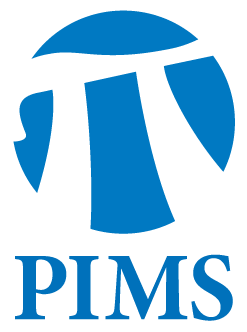## PIMS/SFU Discrete Math Seminar: Irene Pivotto

• Date: 10/11/2011
• Time: 14:30
Lecturer(s):
Irene Pivotto
Location:

Simon Fraser University

Topic:

Pairs of representations of signed binary matroids.

Description:

Abstract: Given a binary matroid $M$ represented by a binary matrix $A$ we can consider the binary matroid represented by $A$ plus an extra binary row$\\Sigma$. We call this a signed matroid and say that $(M,\\Sigma)$ is arepresentation of the signed matroid. We show that for every pair of representations $(M_1,\\Sigma_1)$ and
$(M_2,\\Sigma_2)$ of the same signed matroid there exist rows $\\Gamma_1$ and $\\Gamma_2$ such that $(M^*_1,\\Gamma_1)$ and $(M^*_2,\\Gamma_2)$ are representations of the same signed matroid (where $M^*$ denotes the dual of $M$). An application of this result relates pairs of signed graphs representing the same even cycle matroid to pairs of grafts representing the same even cut matroid. All terms used from matroid theory will be defined during the talk.

This is joint work with B. Guenin and P. Wollan.

Other Information:

Location: K9509